7.4: The van der Waals Equation

Even though van der Waals EOS (vdW EOS) has been around for more than one hundred years, we still recognize van der Waals’ achievements as crucial in revolutionizing our thinking about EOS. We talk about vdW EOS because of pedagogical reasons, not because it finds any practical application in today’s world. In fact, vdW EOS is not used for any practical design purposes. However, most of the EOS being used widely today for practical design purposes have been derived from vdW EOS.

The contributions of vdW EOS can be summarized as follows:

• It radically improved predictive capability over ideal gas EOS,
• It was the first to predict continuity of matter between gas and liquid,
• It formulated the Principle of Corresponding States (PCS),
• It laid foundations for modern cubic EOS.

In his PhD thesis in 1873, van der Waals proposed to semi-empirically remove the main key “weaknesses” that the ideal EOS carried with it. Essentially, what he did was to look again at the basic assumptions that underlie the ideal EOS, which we have listed above.

vdW accounted for the non-zero molecular volume and non-zero force of attraction of a real substance. He realized that there is a point at which the volume occupied by the molecules cannot be neglected. One of the first things vdW recognized is that molecules must have a finite volume, and that volume must be subtracted from the volume of the container. At the same time, he modified the pressure term to acknowledge the fact that molecules do interact with each other though cohesive forces. These are the two main valuable recognitions that he introduced.

The ideal EOS states:(7.5)

or,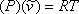(7.6)

whererepresent the molar volume () of the substance.

vdW focused his attention on modifying the terms “P” and “v” in the original ideal gas law by introducing an appropriate correction. Looking back at the inequality of equation (6.4) in Module 6, vdW proposed that the difference of both pressures is the result of the attraction forces — cohesive forces — neglected in the ideal model (equation 7.6) and thus,(7.7)

At this point, vdW postulated the term δPattraction, an inverse function of the mean distance between molecules — this being a direct consequence of Newton’s law of inertial attraction forces, F α (distance)-2. Recognizing that the volume of the gas is a measure of the mean distance between molecules (the smaller the volume, the closer the molecules and vice versa),

(7.8)

and using “a” as a constant of proportionality,(7.9)

Next, vdW took care of the inequality in equation (6.5) (see Module 6). Any particle occupies a physical space; hence, the space available to the gas is less than the total volume of its container. Let us say we can experimentally determine the actual physical space that all the molecules in the container occupy, and that we call it “b”, or the co-volume. vdW then proposed:(7.10)

The inclusion of a parameter “b” (co-volume) recognizes the role of repulsive forces. Repulsive forces prevent molecules from “destroying” one another by not letting them get too close. In a condensed state, there is a maximum allowable “closeness” among molecules. Therefore, it is because of repulsive forces that we cannot compress the volume of a fluid beyond its co-volume value “b”. If the molecules get too close to each other, repulsion forces take over to prevent their self-destruction.

In summary, vdW proposed to correct the pressure and volume terms of the ideal model represented by equation (7.6). The “new” modified-ideal equation of state becomes: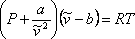(7.11a)

or,(7.11b)
where:
P = absolute pressure
v = molar volume
T = absolute temperature
R = universal gas constant

Equation (7.11b) demonstrates that vdW EOS is explicit in pressure. At this stage it is important to stress that any prediction forfrom equations (7.11) is meaningless due to the physical significance that we have attached to this parameter. Since all the others parameters are constants, equations (7.11) are functional relationships of the variables P, T and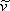:(7.12)

Therefore, equation (7.11a) expresses a PVT relationship and hence, it is an equation of state. In this equation, “a” and “b” are constants that are specific to each component. However, the numerical value of “R” depends on the system of units chosen, as we discussed above.

It is time to ask ourselves a very important question:

How do we calculate “a” and “b” for each substance?

As can be inferred from their definitions, “a” and “b” are different for different substances; i.e.,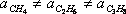;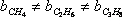How do we relate “a” and “b” to well-known and easily-obtainable physical properties of substances?

It turns out that there are a set of conditions called criticality conditions that must be satisfied for all systems, provided that those systems satisfy the 2nd law of thermodynamics. Indeed, in the previous chapter we recognized that the critical isotherm of a pure substance has a point of inflexion (change of curvature) at the critical point. Furthermore, we recognized the critical point to be the maximum point (apex) of the P-V envelope. This condition of horizontal inflexion of the critical isotherm at the critical point is mathematically imposed by the expression:(7.13)

These conditions are called the criticality conditions. It turns out that when one imposes these conditions on equation (7.11a), one is able to derive expressions for the parameters “a” and “b” as a function of critical properties as follows:(7.14a)(7.14b)

You may want to prove this as an exercise. “a” and “b” can therefore be known because they are functions of known (tabulated) properties of all substances of interest (critical pressure and temperature.)

So far, we have applied vdW EOS to pure components. Can we extrapolate this to apply to multi-component systems?

To extend this concept to a system of more than one component, we use what is called a mixing rule. A mixing rule relates the parameters that characterize the mixture (am and bm) to the individual contributions of the pure components that make up that mixture (ai and bi)

How do we do this? vdW proposed to weight the contributions of each component using their mole compositions, as follows:(7.15a)(7.15b)

The former is called the quadratic mixing rule, while the latter is known as the linear mixing rule.

Contributors

• Prof. Michael Adewumi (The Pennsylvania State University). Some or all of the content of this module was taken from Penn State's College of Earth and Mineral Sciences' OER Initiative.